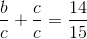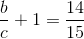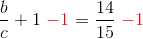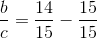## Example Questions

### Example Question #3 : Rational Expressions

If √(ab) = 8, and a= b, what is a?

64

4

2

16

10

4

Explanation:

If we plug in a2 for b in the radical expression, we get √(a3) = 8. This can be rewritten as a3/2 = 8. Thus, loga 8 = 3/2. Plugging in the answer choices gives 4 as the correct answer.

### Example Question #4 : Rational Expressions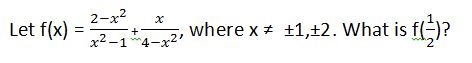–11/5

–37/15

9/5

37/15

–9/5

–11/5

Explanation: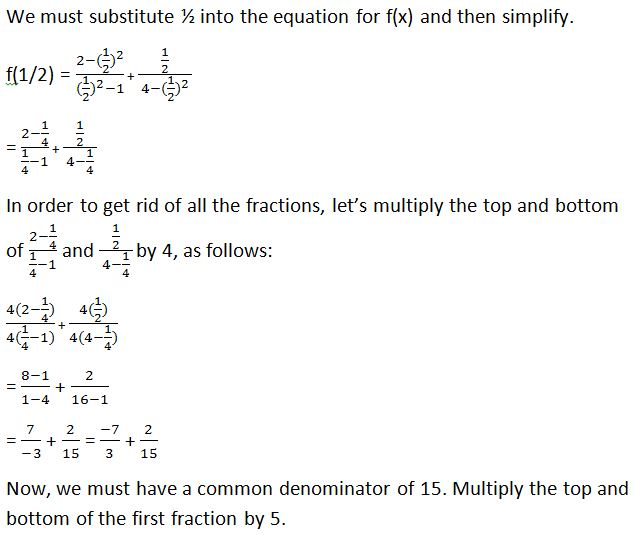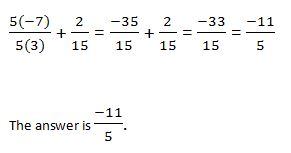### Example Question #5 : Rational Expressions

If Jill walks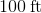in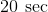, how long will it take Jill to walk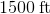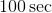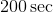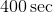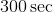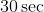Explanation:

To solve this, we need to set a proportion.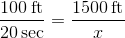Cross Multiply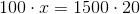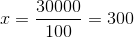So it will take Jillto walk### Example Question #6 : Rational Expressions

If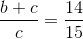, then which of the following must be also true?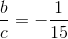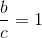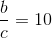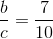Explanation: